text mining with python course Archives - Page 2 of 2 - DexLab Analytics | Big Data Hadoop SAS R Analytics Predictive Modeling & Excel VBA

## Python is the Leader in Data Science: Know WhyFrom being simple and effective to being updated and thereby, solving almost everything that the booming industry of Data Science of today can look up to, Python boasts of it all.

It’s not a shock that Python is finding its uses in an array of industries. It is, in fact, the language that the Data Scientists rely on. Thus, our tailored courses of Python Certification Training in Delhi would be helpful for all in this digital age.

Let’s see some more of the advantages for which Python stands distinguished among the other programming languages:

#### Handling Data without a Hassle

The field of Data Science is entrusted with the handling of incredibly large amounts of data which is found to be intricate to compute. However, with Python, it is now simpler than ever. Any of the other high-level programming languages would make it rather difficult and messy compared to the peerless Python, if we talk about analytical and quantitative computing.

#### Open Source Programming Language

Python is an open-source programming language. Wonder why this programming language is the most preferred still?

It truly opens a whole lot of opportunities that the language can build upon, being open-source in nature. Furthermore, there is not a single restriction regarding Python. Thus, you can be as creative as you wish on this programming language.

#### It is Powerful and Easy to Use

Python is an easy language right from the start for which it has become so popular. Any of the beginners with just the rudimentary knowledge can start fine with Python. Besides, once you are on with this programming language, you can start progressing with it day by day at your own pace.

The implementation of the code has a slower approach in the languages: Java, C and C#, but if you try Python, you would discover that it is fast to debug and effective to perform. The prompt results in coding would aid with an added boost in your work.

#### In the Library of Python

Python is an all-absorbing language that even supports the cutting edge technologies of Machine Learning and Artificial Intelligence. And on top of it, Python also offers its users a colossal database of libraries. Therefore, you can simply check in the libraries, import them and then implement all of them in your day to day coding.

#### It is Highly Scalable

In the parameter of scalability, Python superbly stands out. The programming languages: R and Java certainly falls short in this factor. Thus, with the ease of scalability and quicker turnaround times, data scientists and nearly all of the organisations exploring Data Science, are choosing Python over any other existing languages.#### It is Peerless in Visualisation and Graphics

As the smooth rendering of quality graphics and visualisation is the demand of the age, Python fits in quite comfortably here. With an exhaustive range of options for visualisation, which are simple and efficient, the world of Data Science is rooting for Python.

With all the benefits that you can reap, Python for data analysis is a must, if you want to be absorbed in the industry of Data Science.

.

## Calculating Geometric Mean Using R and Python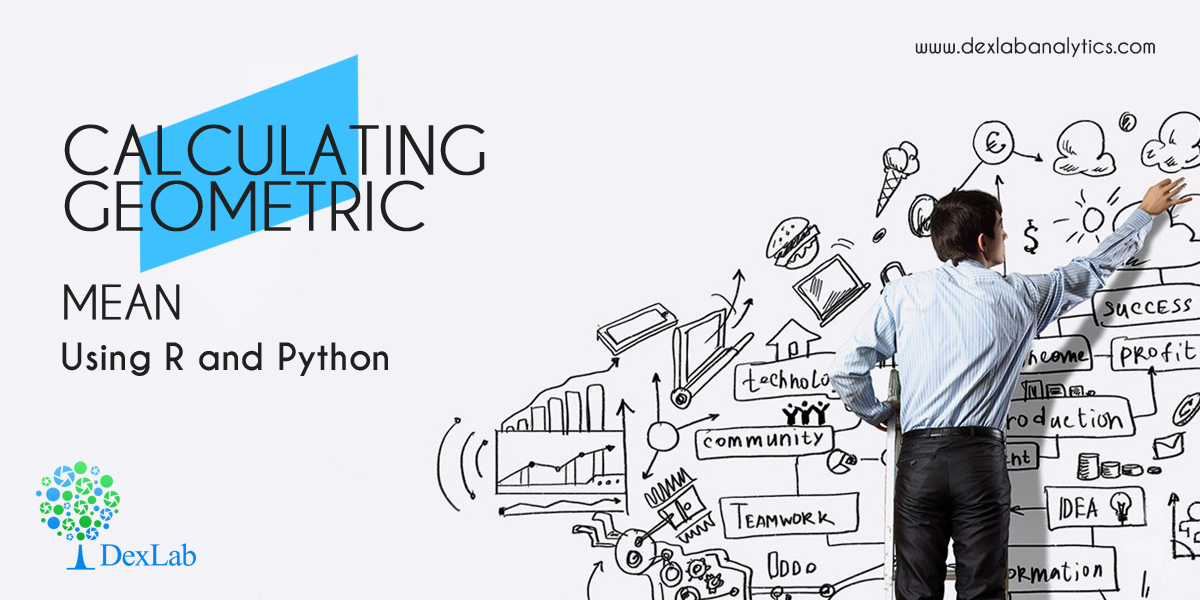In this blog, we are going to discuss the Geometric Mean and its application using Python and R.

Geometric Mean of group of ‘n’ observations is the nth root of their product. It is defined only when all observations have the same sign and none of them is zero.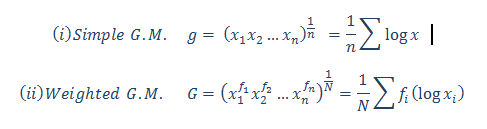#### Calculate the Geometric Mean of the salary increment of 12 employees. From the following table, calculate the average salary increment of the year (2019-2020):-

 Name Salary Increment inPercentage (%) Ritesh 10.09% Heena 15.45% Kritika 9% Anuradha 13.06% Gaurav 20% Prakash 14% Aarti 16% Meena 6.25% Utkarsh 12.85% Chirag 10% Neha 18% Smrita 21.36%

#### Calculate the Geometric Mean in R: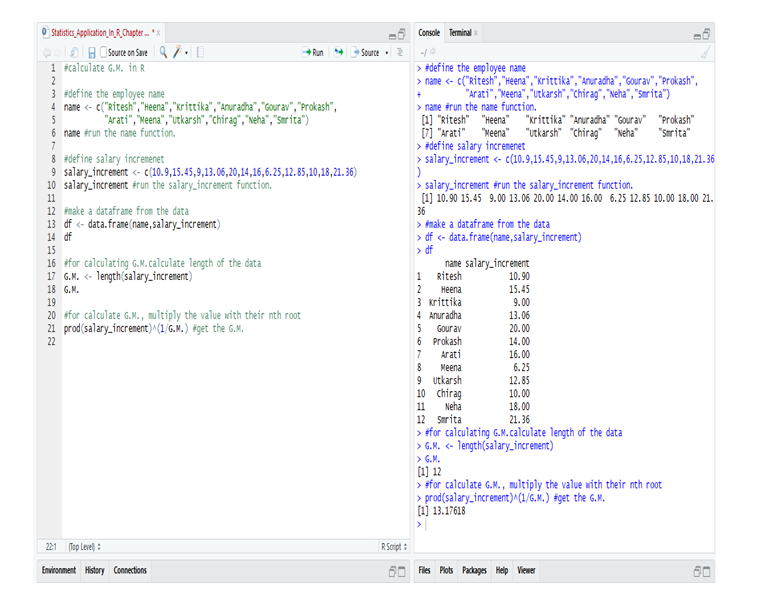So, from the data of the employee’s in R we calculate the G.M. and get that the average salary increment in the year (2019-2020) = 13.17618 or 13.18% (approx).

#### Calculate the Geometric Mean in Python: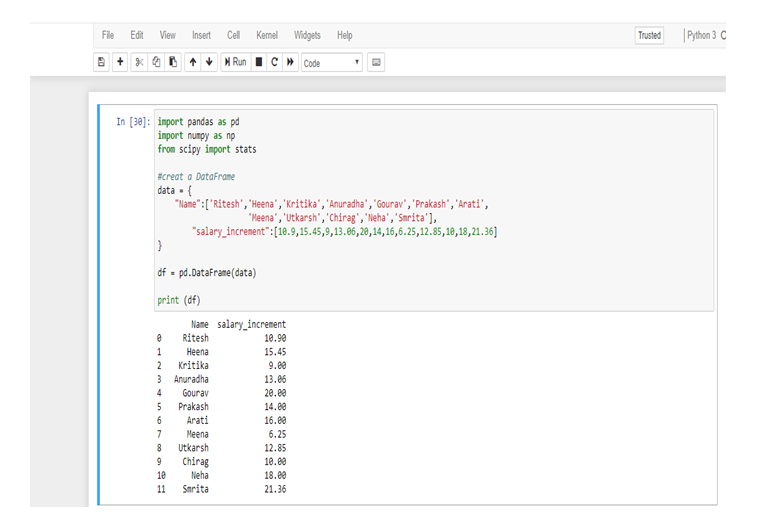First, make a data frame in Python from the following table.

Now, calculate the Geometric Mean from the data-frame.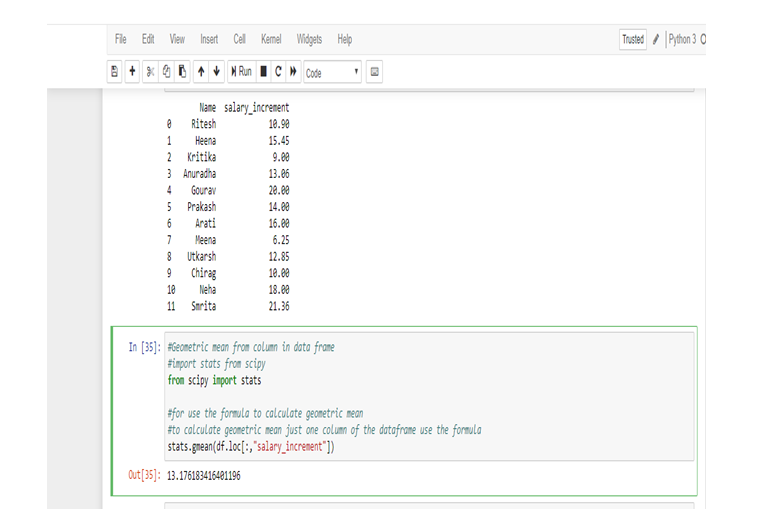So, from the data of the employee’s in Python we calculate the G.M. and get that the average salary increment in the year (2019-2020) = 13.176183416401196 or 13.18% (approx).

We use Geometric Mean for calculating ratios, rates and percentages. And it is not affected by the extreme value or outlier. In this particular problem, we use Geometric Mean because an average of the salary increment of the employee’s not affected by the extreme highest or extreme lowest value, that’s why the salary increment rates of Meena and Smrita do not have any effect on the total average rate.

Geometric Mean gives small value than Arithmetic Mean.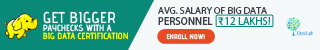Note: This is a continuation of the blog: Statistical Application in R & Python: Chapter 1 – Measure of Central Tendency. It would be better to go through the first installment and then read this one. More blogs are to be followed, so stay tuned.

DexLab Analytics is a premier Python training institute in Delhi. Our industry-relevant courses are carefully crafted by experts. Follow us on Facebook and Instagram.

## Statistical Application In R & Python: Chapter 1 – Measure Of Central Tendency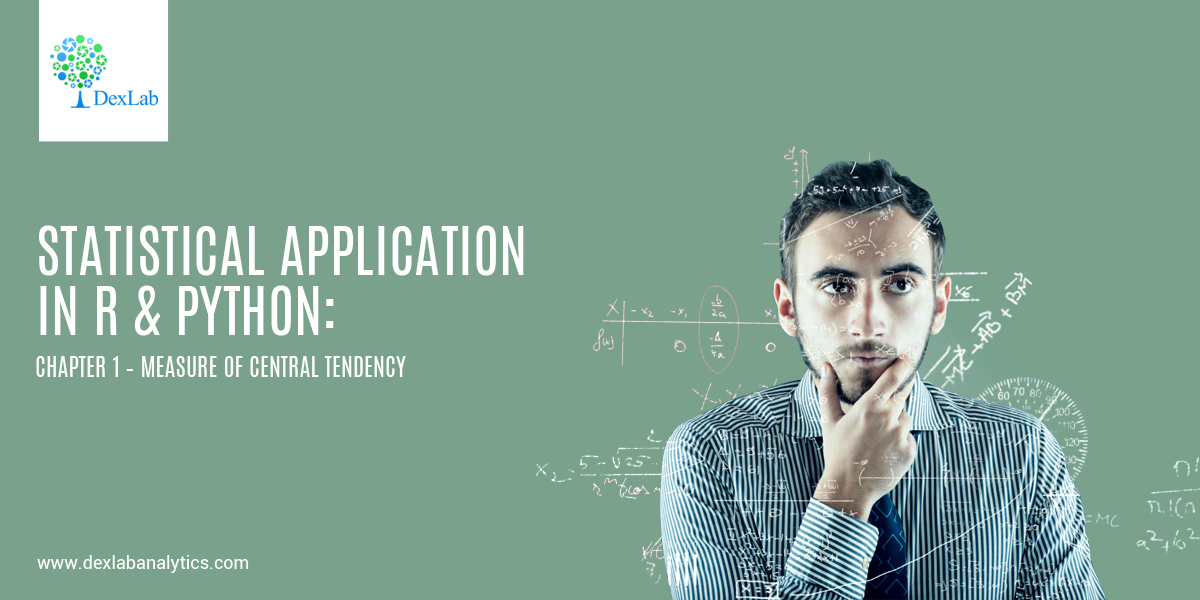Statistical analysis helps explore data relationship and develop high-end models to frame better decisions. It’s an intricate process of collecting and evaluating data to define the nature of data that has to be analyzed.

Below, we dig into the basics of statistical application in R and Python using the measure of central tendency.

• #### Introduction:-

As body methods for the study of numerical data, if some rows or columns are too long, in such cases, it becomes necessary to summarize data in an easily manageable form. The purpose is to serve by classifying the data in the form of frequency distribution and various graphs. When data relate to a variable, the process of summarization can be taken a step further by using certain descriptive measures. The dim is to focus on certain features that are central frequency and description.• #### Central Tendency :

In a set of data, they have a tendency, notwithstanding their variability, to cluster-around a central value and the tendency of the quantitative statistical observations is called central tendency.

The three measures of the central tendency are commonly used is:-

• Mean
• Median
• Mode

The description of these 3 estimators start below:-

• #### Mean:-

Mean is the average of central tendency and is the most commonly used measures.

The concept of mean is divided into three parts:-

• Arithmetic mean.
• Geometric mean.
• Harmonic mean.

Mainly the mean refers to an arithmetic mean.

• #### Arithmetic Mean (A.M.):-

The arithmetic mean of a set of observations is defined to be their sum, divided by the number of observations.

For n numbers of observation (x1,x2,… ,xn )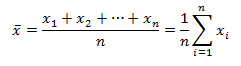• #### Weighted A.M.

For frequency distribution where  have  frequencies. (i=1,2,3…)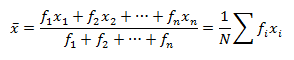• #### Application of A.M.:-

Let’s, calculate the mean of Age, Height & Weight from the given data.

 Name Sex Age Height Weight Ritesh M 24 6.9 112.5 Heena F 23 5.65 84 Kritika F 23 6.53 98 Anuradha F 24 6.28 102.5 Gaurav M 24 6.35 102.5 Prakash M 22 5.73 83 Aarti F 22 5.98 84.5 Meena F 25 6.25 112.5 Utkarsh M 23 6.25 84 Chirag M 22 5.9 99.5 Neha F 21 5.13 50.5 Smrita F 24 6.43 90

#### Calculating Mean in Python: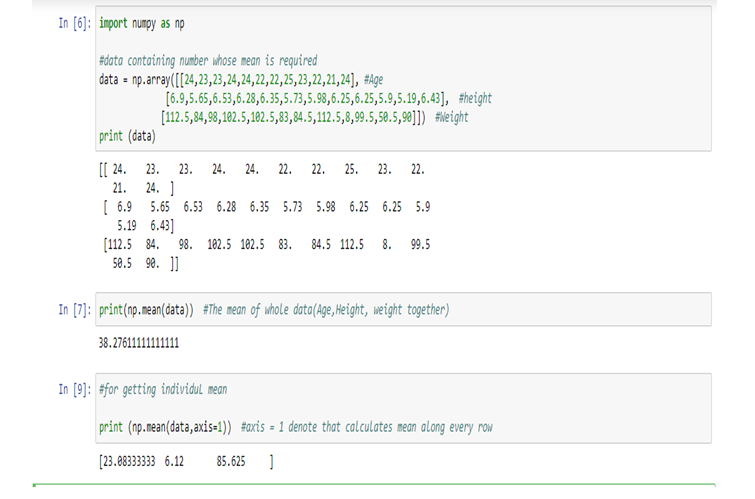Therefore,

Age (Mean) = 23.08333333, Height (Mean) = 6.12, weight(Mean) = 85.625

#### Calculating Mean in R: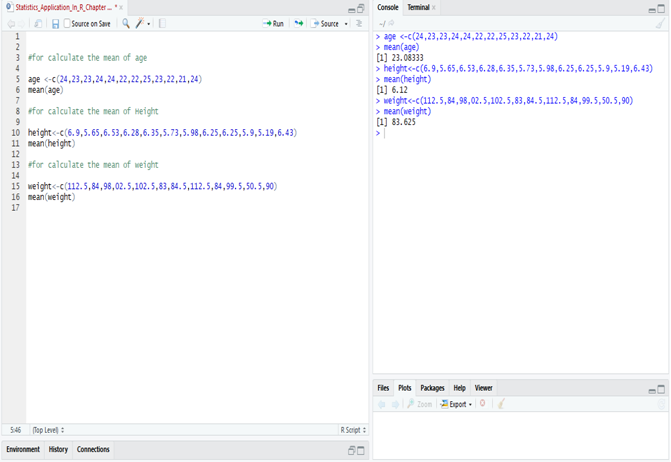• #### Application of Weighted A.M.:-

The weighted mean is denoted that the mean with frequency.

#### Calculate the average price per ton of coal purchased by the industry for the half-year.

 Month Price Per Ton Tons Purchased January Rs. 52.49 26 February Rs. 62.23 34 March Rs. 87.26 40 April Rs. 45.25 54 May Rs. 78.56 13 June Rs. 69.25 45

#### Data to solve:

 Month Price (Rs)Per Ton(x) TonsPurchased(f) fx=y(Main Data) January 52.49 26 1364.74 February 62.23 34 2115.82 March 87.26 40 3490.4 April 45.25 54 2443.5 May 78.56 13 1021.28 June 69.25 45 3116.25 Total 395.04 N=212 13551.99

The price is denoted as x (52.49, 62.23, 87.26, 45.25, 78.56, 69.25 [in Rs.])=395.04

The amount of purchased (frequency) is denoted by f (26, 34, 40, 54, 13, 45) = 212 (N)

Then multiply the x and f and we get the total amount which is denoted by y, fx(y) = 13551.99

#### Calculate Weighted Mean in R: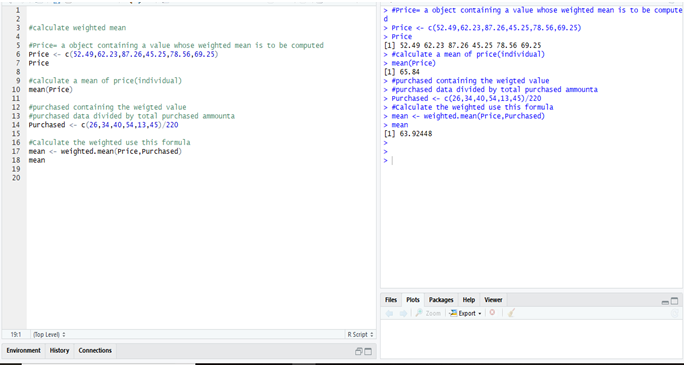#### Calculate Weighted Mean in Python: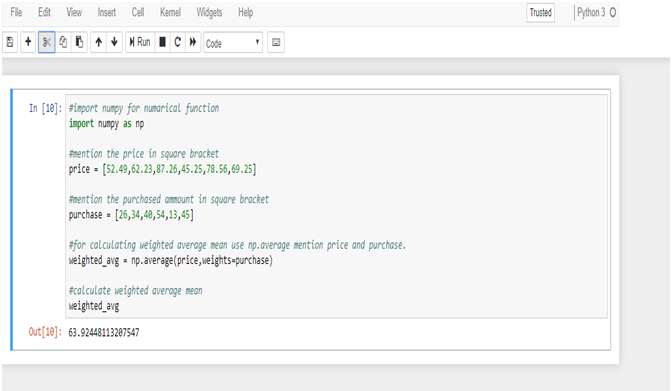To calculate the weighted mean from R & Python we get the same result = 63.9244811.

Want to know more about the nature of data? Keen to perform high-end statistical analysis using Python and R? Follow DexLab Analytics, an excellent Python training center in Gurgaon, India. Our team of consultants will help you learn the basics of R and Python in the easiest manner possible.